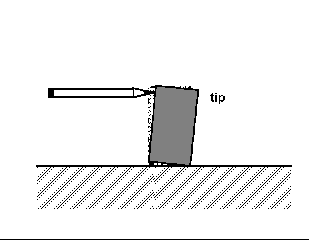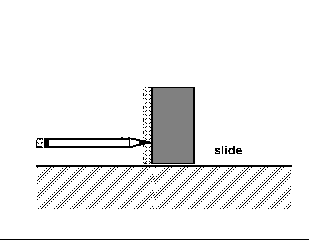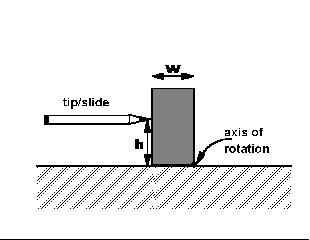# Experiment of The Month

## Measuring Friction with Pencil and a Ruler

The MU Physics Department does not claim to have invented these labs. The origin of these labs is currently unknown to us. Our labs do not have written instructions. In keeping with this spirit, the description given here will be brief and general. The intent is that each performance of the lab will be unique; in each nature will reveal a slightly different face to the observer.

This experiment was introduced to us by Dr. Miziumski. As with many of our labs, this exercise imitates the actual process of doing physics: We make a measurement, a prediction based on analysis of a model of the measurement, and a new measurement to test the prediction (and thereby test the model).

A short (10 to 20 cm long) piece of "2x4" lumber is placed on a flat piece of wood, as shown. When the pencil is used to push (near the top) on the wood, the piece tips over. When a pencil is used to push (near the bottom) on the wood, the wood slides (before it tips over) when the force by the pencil "overcomes" the maximum static friction force. We are interested in the "just-right" condition, when the pencil is at height h. If the pencil is moved up, the block tips when pushed. If the pencil is moved down, the block slides when pushed.

When the block is about to tip, all the force by the table is exerted at the axis of rotation, shown in the lowest figure. Taking this axis of rotation, the analysis uses both vertical and horizontal components of the force equilibrium condition (Ftotal=0) and the single component of the torque equilibrium condition. The result is that the coefficient of static friction is given by W/(2h).

A quicker derivation of this result comes from taking the place where the pencil point touches the block as the center of rotation.

This coefficient of friction is measured for several faces of the 2x4. In each case, the error is calculated from the estimated error in the two length measurements. The several values may or may not agree with uncertainty, depending on the details of the wood surfaces.

Now the prediction is made: Using the measured friction coefficient, students predict the tangent of the angle at which the block will slip when the board on which it sits is slowly tilted up from horizontal. They measure the tangent of this angle and compare the observations to the prediction. Often, but not always, the prediction agrees with the observation, within experimental uncertainty## Experiment Of The Month

View past labs experiments.